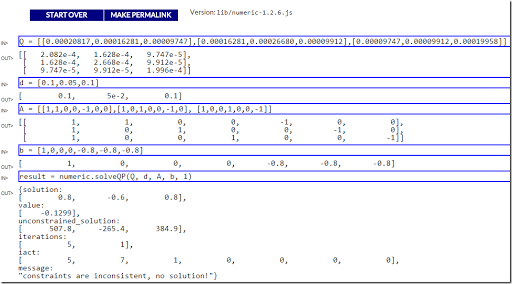## Friday, February 26, 2016

### Quadprog: R vs Javascript

##### R

The R package quadprog contains a QP (Quadratic Programming) algorithm that solves the following problem:

 \begin{align} \min\>&\frac{1}{2}x^TQx-d^Tx \\ &A_1^Tx = b_1 \\ &A_2^Tx \ge b_2 \end{align}

The funny factor $$\frac{1}{2}$$ is often used in QP algorithms. I suspect this is largely for historical reasons (the background may be that the gradients are then just the $$q_{i,j}$$ values).  Notice in the input that the first $$m_1$$ constraints are equality constraints and the next $$m_2$$ constraints are $$\ge$$ inequalities. Bounds need to be represented as constraints and $$\le$$ constraints $$a^Tx \le b$$ need to be passed on as $$-a^Tx \ge -b$$. The fact that linear equations are expressed as $$A^Tx = b$$ is unusual ($$Ax = b$$ is far more conventional).

Here is a small example on how one can use it to solve a small portfolio problem:

 \begin{align} \min\>&\frac{1}{2}x^TQx-\lambda d^Tx \\ & \sum_i x_i=1 \\ & 0 \le x_i \le 0.8 \end{align}

where we assume $$\lambda=1$$.

The R code can look like:

> library(quadprog)
> Q <- rbind(c(0.00020817,0.00016281,0.00009747),
+           c(0.00016281,0.00026680,0.00009912),
+           c(0.00009747,0.00009912,0.00019958))
> Q
[,1]       [,2]       [,3]
[1,] 0.00020817 0.00016281 0.00009747
[2,] 0.00016281 0.00026680 0.00009912
[3,] 0.00009747 0.00009912 0.00019958
> d = c(0.1,0.05,0.1)
> d
 0.10 0.05 0.10
> A = rbind(c(1,1,0,0,-1,0,0),
+           c(1,0,1,0,0,-1,0),
+           c(1,0,0,1,0,0,-1))
> A
[,1] [,2] [,3] [,4] [,5] [,6] [,7]
[1,]    1    1    0    0   -1    0    0
[2,]    1    0    1    0    0   -1    0
[3,]    1    0    0    1    0    0   -1
> b = c(1,0,0,0,-0.8,-0.8,-0.8)
> b
  1.0  0.0  0.0  0.0 -0.8 -0.8 -0.8
> solve.QP(Q,d,A,b,1)
$solution  0.4798177 0.0000000 0.5201823$value
 -0.09992471

$unconstrained.solution  507.7568 -265.4413 384.9057$iterations
 6 3

$Lagrangian  0.09984941 0.00000000 0.04997909 0.00000000 0.00000000 0.00000000 0.00000000$iact
 1 3
##### Javascript

Here we try the javascript version of this algorithm, found here: www.numericjs.com.  We throw the same data at it. We use the notebook-like interface to enter the data and solve the problem:Looks like the constraints cause some problems here. The unconstrained solution is the same. But when the constraints are added to the problem the algorithm fails miserably. At least on this problem, R seems to be a winner.

##### Matlab

Matlab also contains a QP algorithm called quadprog, documented here. The usage is a little bit more natural in my opinion: they don’t transpose A and they allow lower- and upper-bounds to be specified directly.

##### References

D. Goldfarb and A. Idnani (1982). Dual and Primal-Dual Methods for Solving Strictly Convex Quadratic Programs. In J. P. Hennart (ed.), Numerical Analysis, Springer-Verlag, Berlin, pages 226–239.

D. Goldfarb and A. Idnani (1983). A numerically stable dual method for solving strictly convex quadratic programs. Mathematical Programming, 27, 1–33